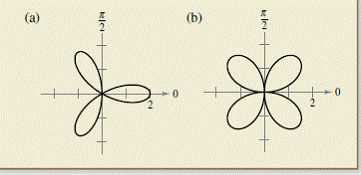Chapter 10.5, Problem 72E

Chapter
Section
Textbook Problem

# HOW DO YOU SEE IT? Which graph, traced out only once, has a larger arc length? Explain your reasoning.To determine

To Calculate: Which of the given graph is traced out only once and has larger arc length.

Explanation

Given:

Graphs are given which are shown as below:

Formula Used:

L=0πf(θ)2+f'(θ)2

Calculation:

Equation of rose curve is given by: r=2cos3θ

In the above graph θ increases from 0 to π

L=0πf(θ)2+f'(θ)2=0π(2cos3θ)2+(6sin3θ)2=0π(4cos23θ)+(36sin23θ)=0π(1+(32sin23θ)

Further solve and get,

L=0π(16cos6θ+(16).dθ

Put cos6θ+1=t2.

Now,

=4202tdt6sin6θ=4202t2dt6(2t2t4)=402dt3(2t2)=4302dt((2)2t2)

### Still sussing out bartleby?

Check out a sample textbook solution.

See a sample solution

#### The Solution to Your Study Problems

Bartleby provides explanations to thousands of textbook problems written by our experts, many with advanced degrees!

Get Started

#### In Exercises 19-24, find the functions f + g, f g, fg, and fg. 24. f(x) = x2 + 1; g(x) = x+1

Applied Calculus for the Managerial, Life, and Social Sciences: A Brief Approach

#### In Problems 33 – 38, solve each inequality. 36.

Mathematical Applications for the Management, Life, and Social Sciences

#### Divide: (42x2y3)(14x2y4)

Elementary Technical Mathematics

#### Evaluate the integral. 11x100dx

Single Variable Calculus: Early Transcendentals

#### The graph of θ = 2 in polar coordinates is a: circle line spiral 3-leaved rose

Study Guide for Stewart's Single Variable Calculus: Early Transcendentals, 8th

#### True or False: By the Integral Test, converges.

Study Guide for Stewart's Multivariable Calculus, 8th

#### Define content analysis and archival research.

Research Methods for the Behavioral Sciences (MindTap Course List)